# machine-learning-conceptsWhat is Big Data? Types, Characteristics and Examples

In this blog, we have discussed the idea of Big Data, which addresses the challenge of managing vast amounts of complex and diverse data. We discussed what constitutes Big Data, its types, characteristics, examples, use cases, the various technologies used, advantages, and the challenges faced by engineers working with Big Data.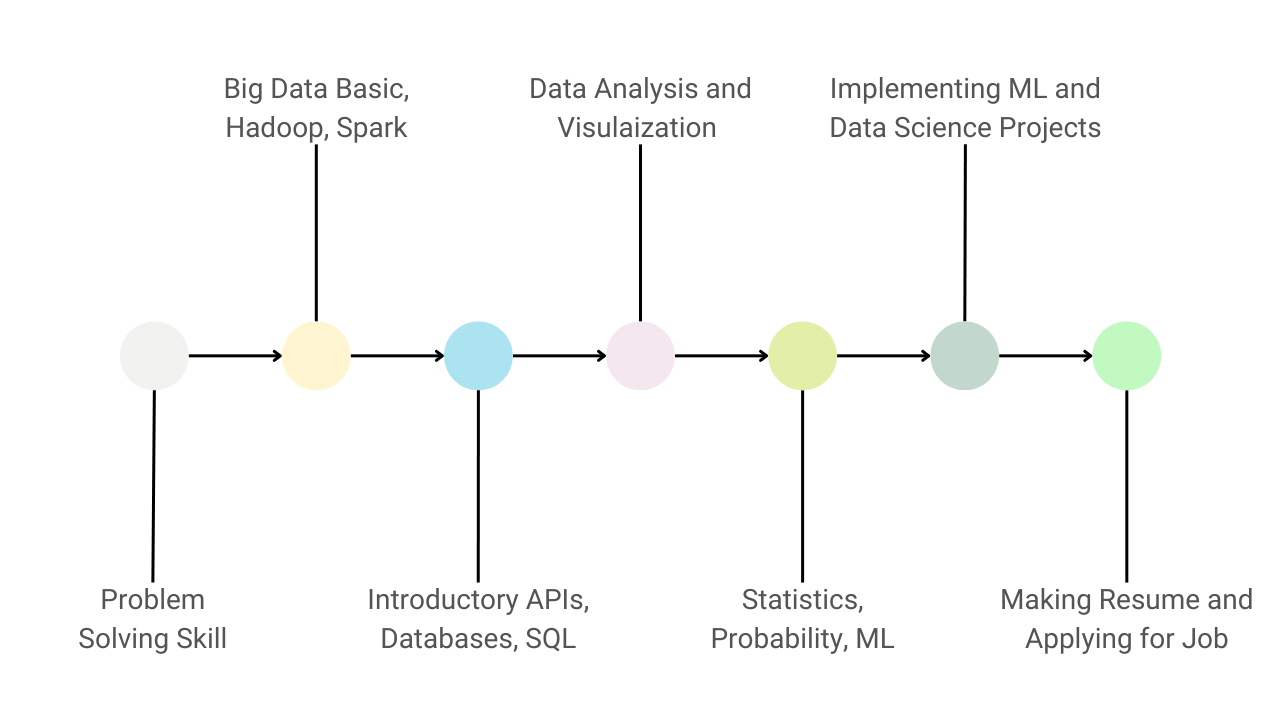Guide to Learn Data Science and Become a Data Scientist

Here are 7 key steps to master data science: 1) Learning Python 2) Understanding big data frameworks like Hadoop and PySpark 3) Learning concepts of APIs, databases and SQL 4) Hands-on experience in data analysis and visualization 5) Learning statistics, probability, and machine learning 6) Building data science projects 7) Making resume and applying for data scientist positions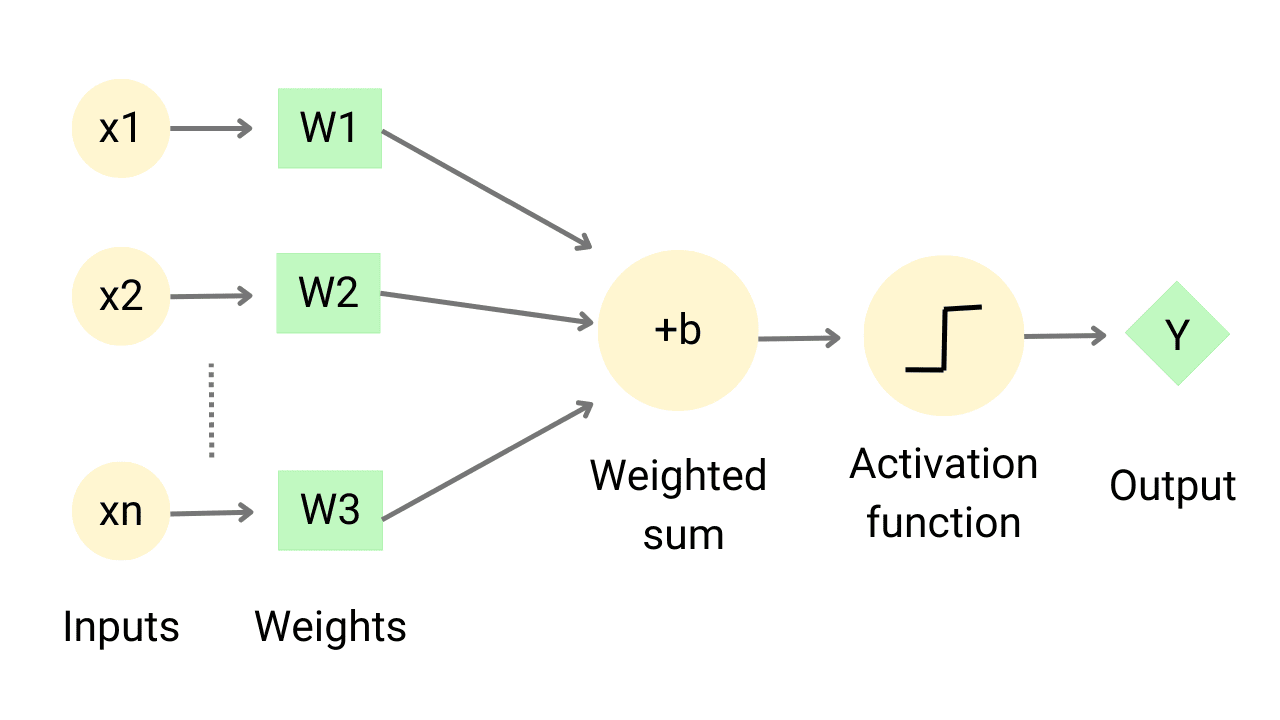Design Perceptron to Learn AND, OR and XOR Logic Gates

Perceptron is the most fundamental unit of Neural Network architecture in Machine Learning. In this article, we will learn to design a perceptron from scratch in Python to make it learn the properties of AND, OR and XOR logic gates. We will observe the need for multiple layer perceptron (MLP) over single layer perceptron.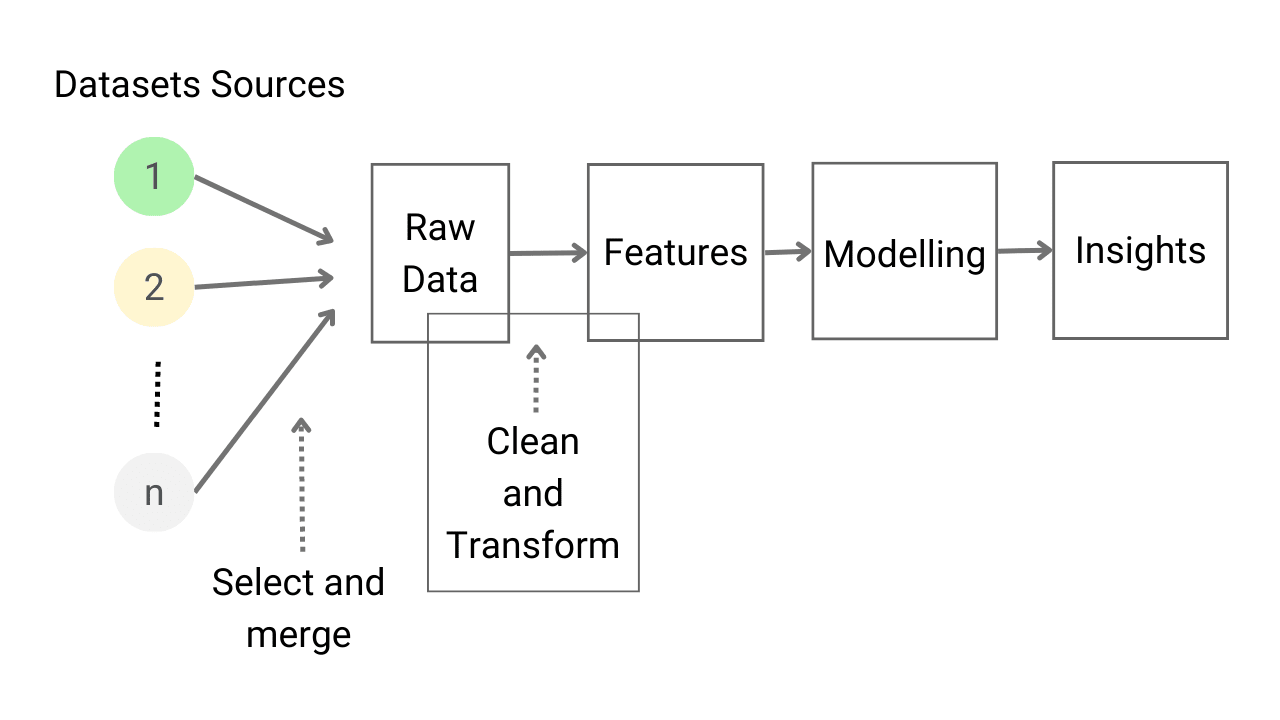Introduction to Feature Engineering for Machine Learning

Feature engineering is the process of selecting, correcting, and generating new features from existing attributes in a dataset to optimize the performance of machine learning models. The main steps involved are feature creation, transformation, extraction, and selection. To improve results, we can employ techniques such as imputation, transformation, scaling, and encoding, which will be discussed in this blog.Data Types and Variables in Python Programming

Python is the most preferred language for developing machine learning and data science applications. It has a large community support that can help debug the errors and resolve all the roadblocks appearing while developing any solution. In this blog, we have discussed various data types like integers, floats, boolean and strings along with their usage in Machine Learning and Data Science.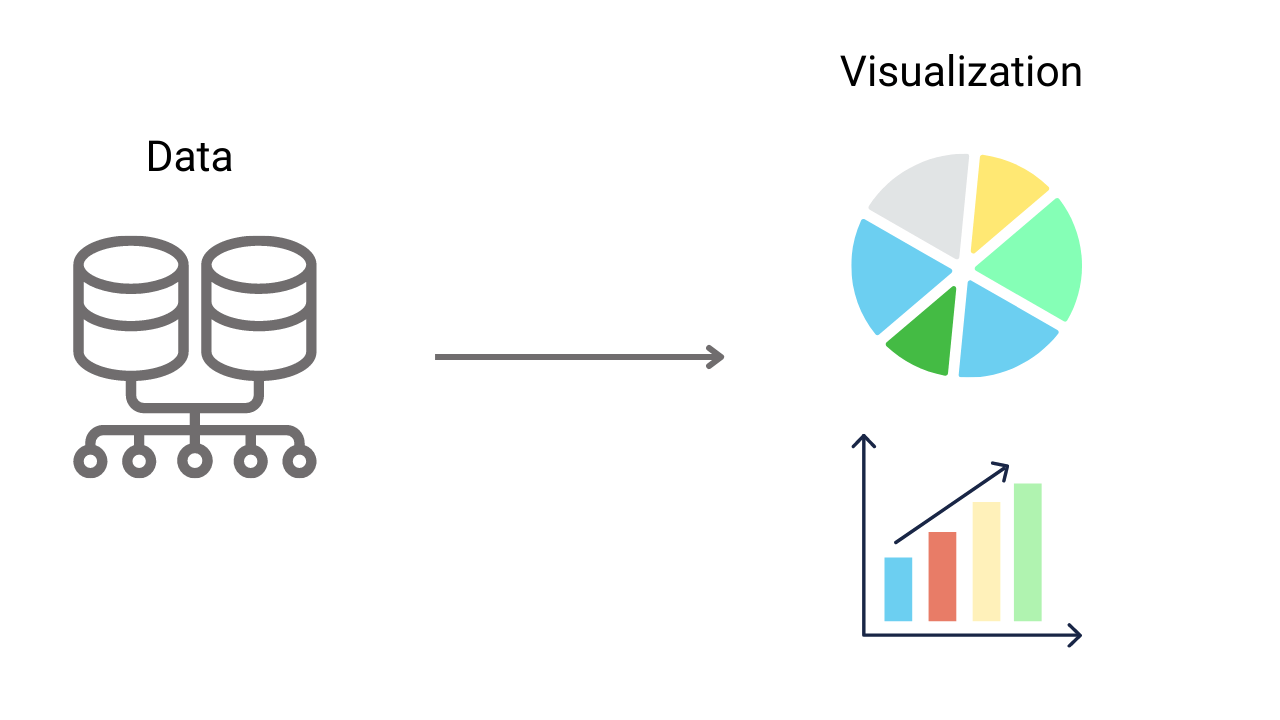Data Visualization Introduction In Data Science

Data Visualization is a technique for presenting information using visual elements, making it accessible and easy to comprehend. It plays a crucial role in all various stages of machine learning and data science. To be effective, data visualizations should be aesthetically simple, creative and informative. In this blog, we explore various processes and examples of data visualization.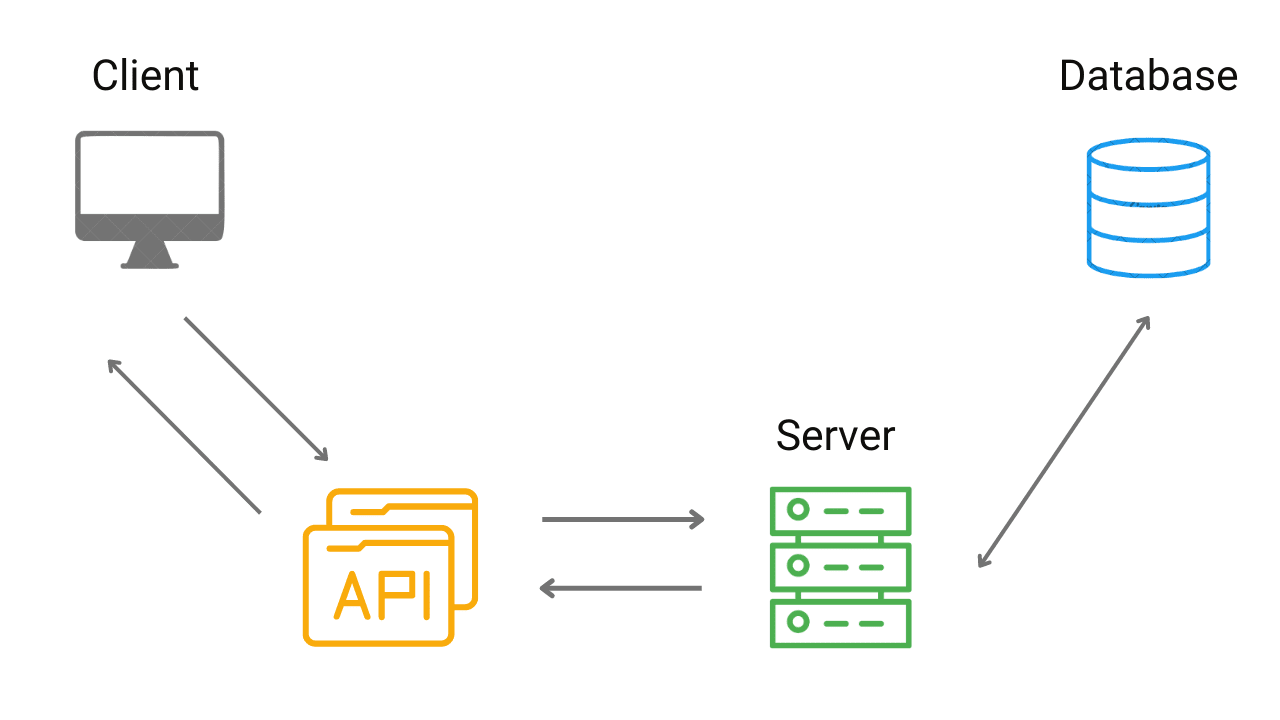Introduction to APIs for Data Science and Machine Learning

In machine learning and data science, API (Application Programming Interface) is a powerful tool that enables seamless communication and data sharing between applications and servers. APIs are mainly used for data gathering and model deployment In data science and ML. This blog provides a step-by-step explanation of how APIs work.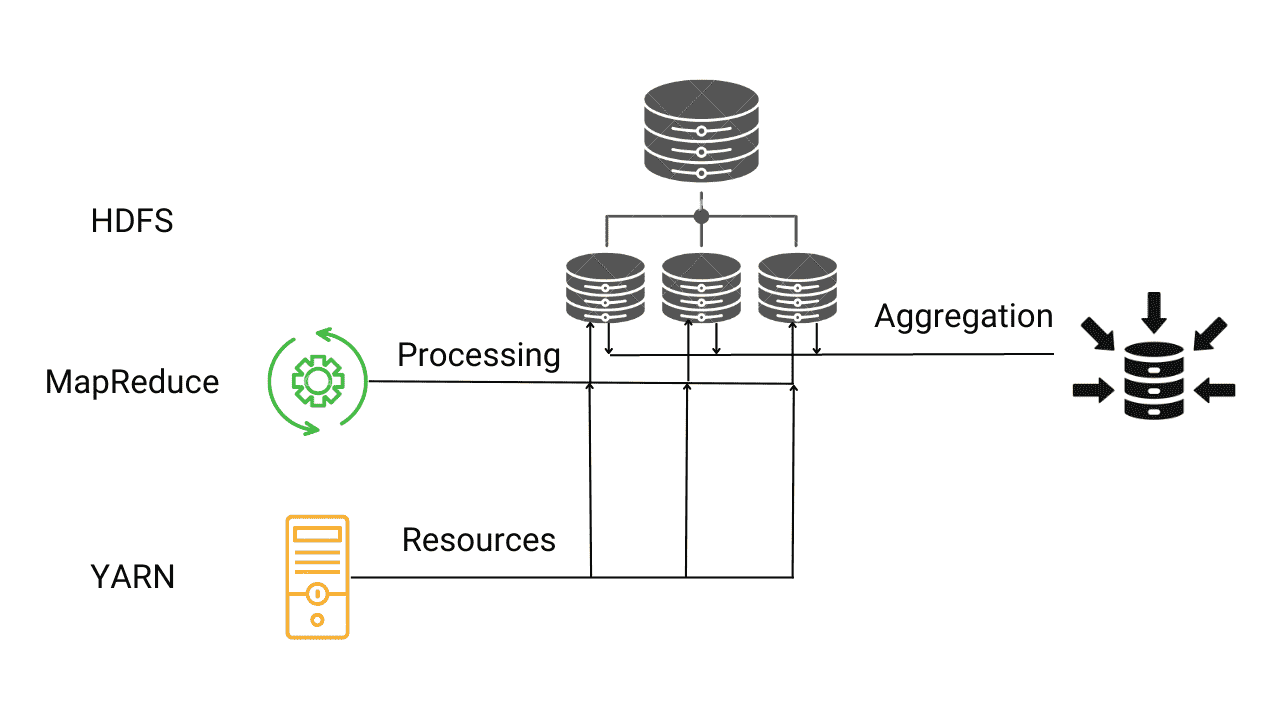Introduction to Hadoop Framework for Big Data

Hadoop is an open-source framework that addresses the analytical and operational needs of Big Data by overcoming the limitations of traditional data analysis methods. With support for highly scalable and fault-tolerant distributed file systems, it allows for parallel processing. It comprises four main components - HDFS, YARN, MapReduce, and Hadoop Common.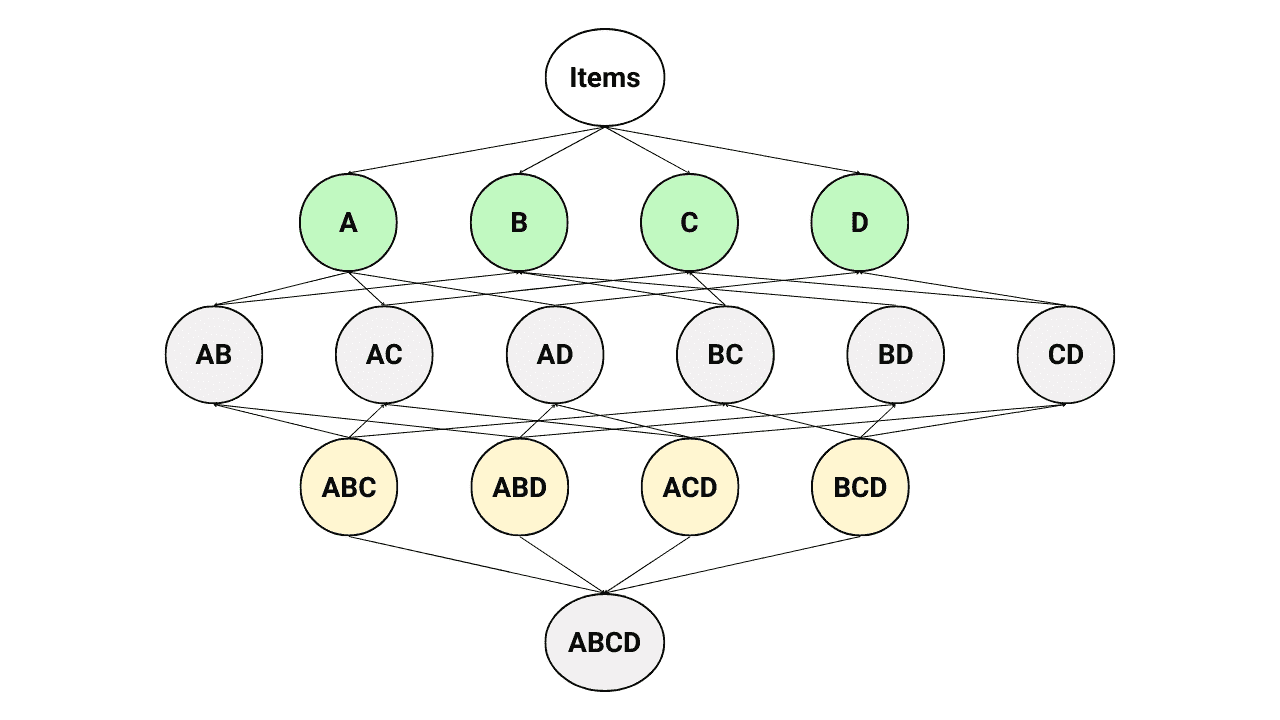What is Apriori Algorithm? Examples and Implementation

The Apriori Algorithm is a powerful tool in association rule mining that helps to uncover the relationships and associations among items. This technique is widely used by supermarkets and online shopping platforms to optimize product placement and offer discounts on bundled purchases. In this article, we have explained its step-by-step functioning and detailed implementation in Python.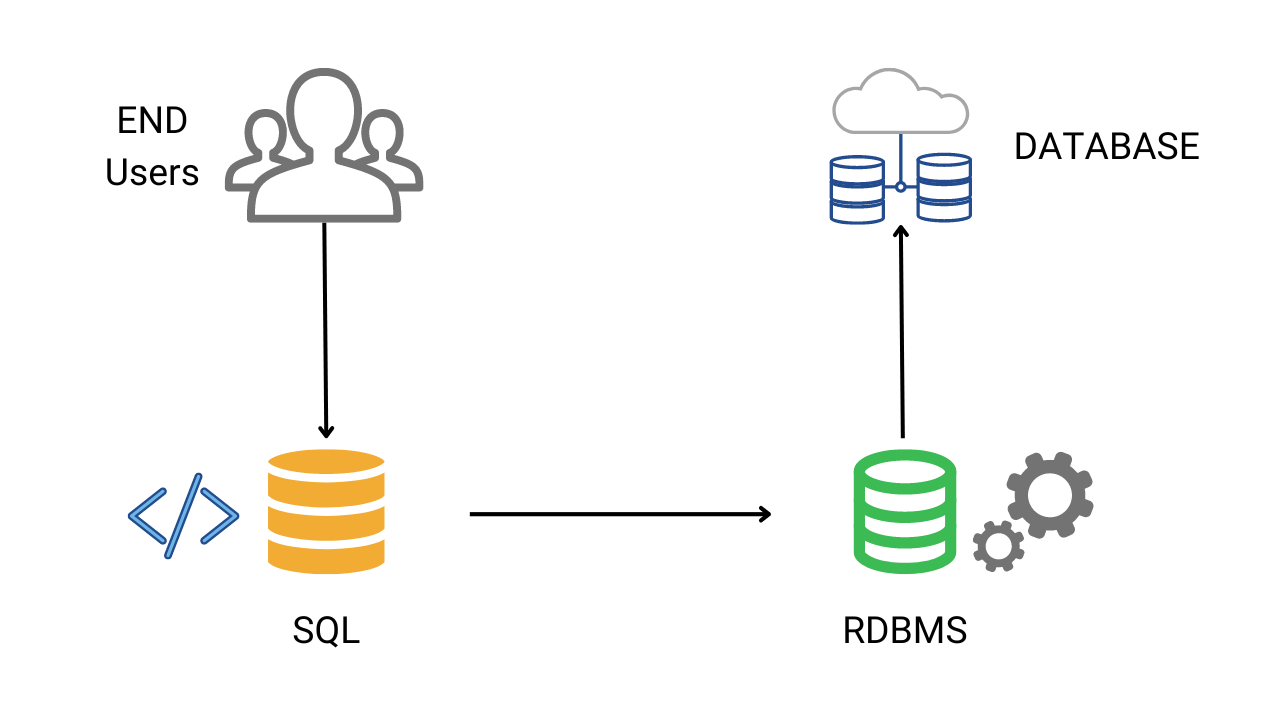Introduction to SQL for Data Science

Based on Reports, more than 40% of data science jobs require SQL as an essential skill. So, to analyze datasets effectively in data science, one should master RDBMS, data cleaning processes, and SQL commands. The major advantage of using SQL is that SQL queries can be executed easily in Python by establishing connections to the database.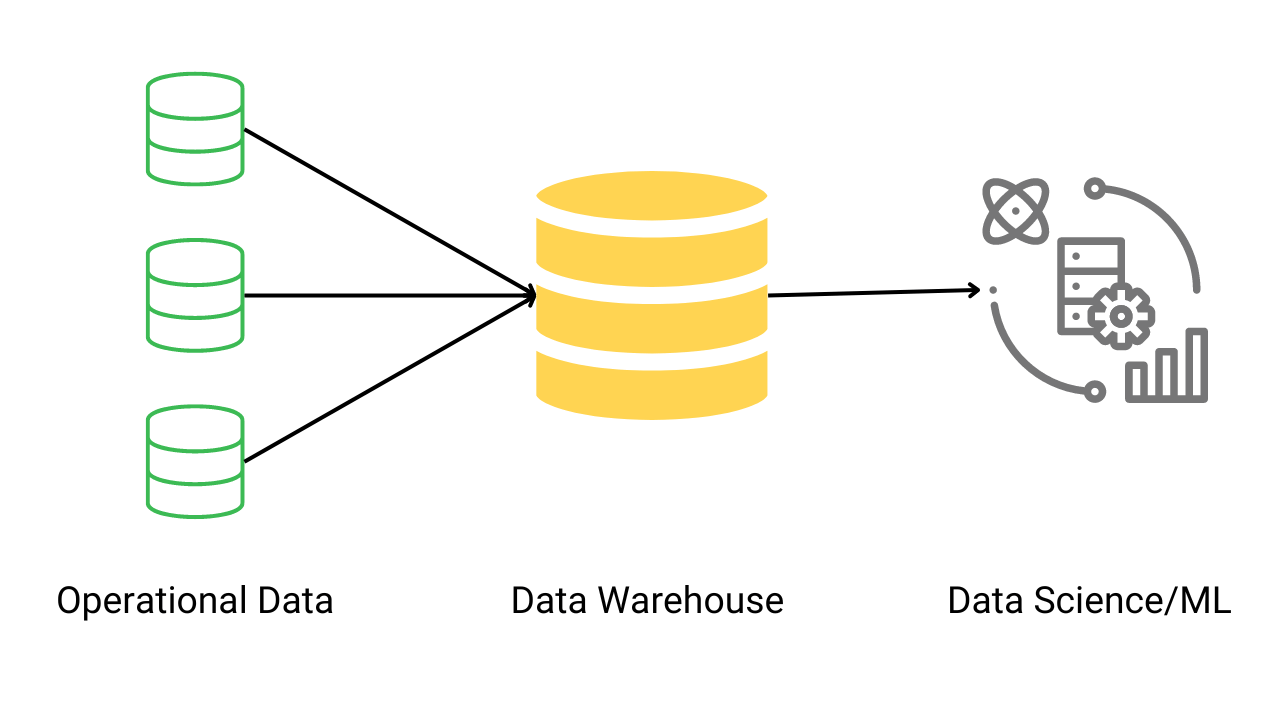Introduction to Databases for Data Science

In data science, databases play a crucial role in storing, managing, and scaling large amounts of data. This data is then analyzed to gain meaningful insights. In this blog, we will delve into the concept of databases and understand how data science relies on them, as well as their advantages.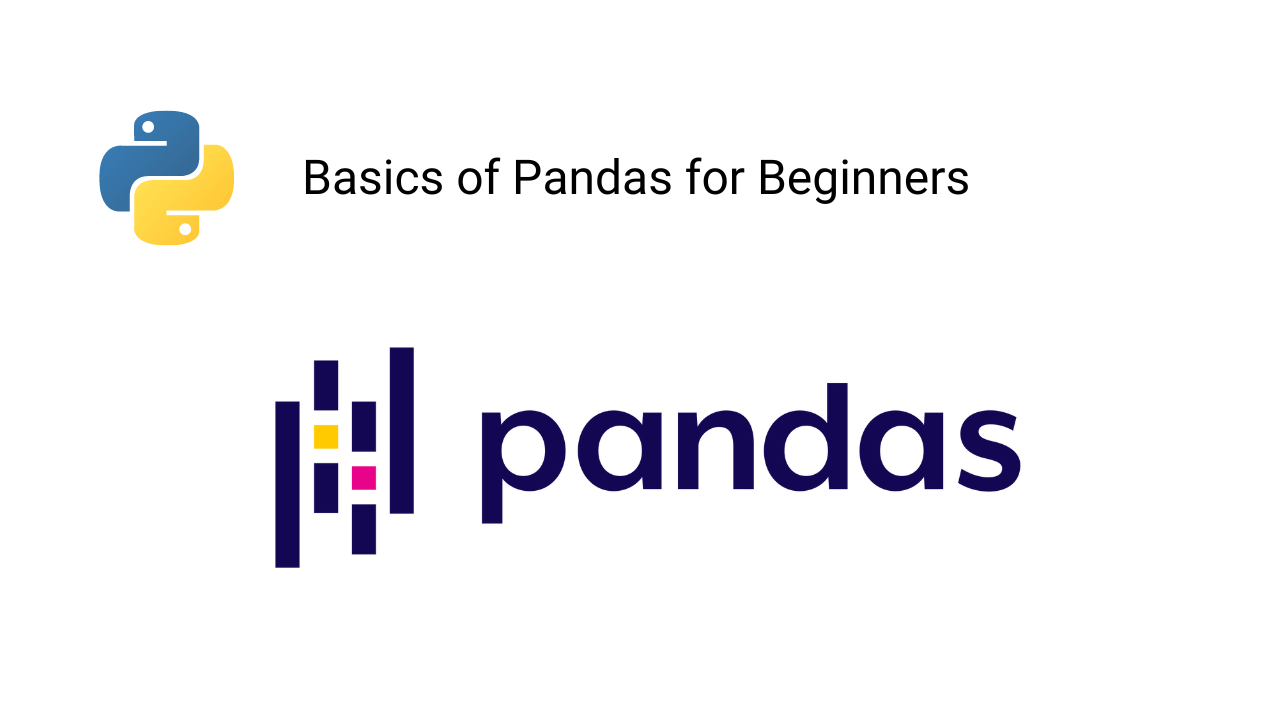Introduction to Pandas Library in Python

Pandas is a widely used Python library by data scientists for managing, processing, and analyzing data. This library offers various efficient tools and functions to streamline data manipulation and analysis. In this blog, we will guide you through the installation process and provide an overview of the frequently used basic Pandas functions in machine learning projects.Introduction to NumPy in Python

Numpy is one of the most essential Python libraries for building machine learning and data science applications. Its ability to perform parallel computing and execute certain functions using C programming makes it lightning-fast. In this blog, we will cover the basics of the Numpy library, including installation and the most commonly used functions for executing mathematical operations.Different Types of Machine Learning Algorithms

Having a clear understanding of the different types of machine learning algorithms is crucial for the success of a machine learning project. Each algorithm has its own strengths, weaknesses, and areas of applicability. Understanding these differences helps to select the most appropriate ML algorithm for a given problem and avoid common mistakes.Gradient Descent Algorithm in Machine Learning

Gradient descent in machine learning is a basic cost function optimization algorithm. In this blog, we have discussed: 1) Limitations of computations in machine learning. 2) What are optimization algorithms, and why do we need them? 3) What is the problem with multiple minima in the cost function? 4) What is gradient descent and how does it work?Introduction to Artificial Neural Networks

Artificial Neural Networks, also known as Neural Networks, is a type of supervised learning algorithm that can be used to solve both classification and regression problems. In this blog, we will discuss the terms used to define a Neural Network, its components, the advantages and disadvantages of Neural Networks compared to other machine learning algorithms, and its use cases.Components of an Artificial Neural Network

To fully grasp the concept of a Neural Network, we need to understand the various components that make up a Neural Network. In this blog, we delve into the key components of a Neural Network, including Neurons, Input Layers, Output Layers, Hidden Layers, Connections, Parameters, Activation Functions, Optimization Algorithms, and Cost Functions. These components work together to solve both classification and regression problems in Machine Learning.What is Data Science? Introduction and Importance

These days, companies are collecting huge amounts of data, but the question is: what is the purpose of this data? This is where data science comes into play. Data science is a field that extracts, processes, analyzes, and interprets data to derive various insights from it. In this blog, we will discuss the importance of data science and various key concepts.KNN Algorithm in Machine Learning

K-Nearest Neighbor is a supervised learning algorithm that can be used to solve classification and regression problems. This algorithm learns without explicitly mapping input variables to the target variables. It is probably the first machine learning algorithm, and due to its simplicity, it is still accepted in solving many industrial problems.Introduction to Scikit-Learn

Scikit-learn is a free machine learning framework available for Python, providing an interface for supervised and unsupervised learning. It is built over the SciPy library and provides every feature catering to every ML requirement. In this blog, we will learn the essential concepts, tools, and features related to Scikit-learn.Feature Selection Techniques in ML

The best machine learninmodel would have the lowest number of features involved in the analysis keeping the performance high. Therefore, determining the relevant features for the model building phase is necessary. In this session, we will see some feature selection methods and discuss the pros and cons of each.Introduction to Feature Scaling in Machine Learning

In this article, we will learn about methods used for scaling different attributes present in our data. Normalization and Standardization are two most used techniques available for scaling features and bring them on same range.It avoids the cases of biases towards higher or lower magnitude features.Regularization in Machine Learning

Regularization is the technique that is used to solve the problem of overfitting in machine learning. In this blog, we have discussed famous machine learning concepts like underfitting, overfitting, accurate fitting, regularization and how it cures overfitting. Mathematical logic behind regularization and difference between L1 and L2 regularization.Evaluation Metrics for Classification Models in ML

Classification problems are among the most used problem statements in machine learning. We evaluate classification models using standard evaluation metrics like confusion matrix, accuracy, precision, recall, ROC and the AUC curves. In this article, we will discuss all these popular evaluation metrics to evaluate the classification models along with their inbuilt functions present in Scikit-learn.Linear Regression in Machine Learning

Linear Regression is a supervised machine learning algorithm used to solve regression problems. In this blog, we have discussed: 1) What is linear regression? 2) Various types 3) The loss function for linear regression 4) Ordinary Least Squares (OLS) method 5) Polynomial regression 6) Python implementation of linear regression.Evaluation Metrics for Regression Models

We evaluate the performance of our regression models in Machine learning using standard metrics. In this article, we will be discussing all the standard evaluation metrics namely MAE, MAPE, MSE, RMSE, R-Squared and Adjusted R-Squared, for regression models used to compare two different models on the same dataset.Customer Segmentation using Machine Learning

Customer segmentation is a machine learning application that involves grouping customers based on similarities in their behaviour. This unsupervised learning technique helps companies create customer groups for targeted marketing. One way to group customers is through hierarchical clustering, which can be visualized using dendrograms. In this blog post, we will demonstrate how to implement hierarchical clustering using Python.Logistic Regression in Machine Learning

Logistic Regression is one of the most used machine learning algorithms. It is a supervised learning algorithm where target variables should be categorical, such as positive or negative, Type A, B, or C, etc. Although the name contains the term "regression", we can also say that it can only solve classification problems.Loss and Cost Function in Machine Learning

Optimization of error function is the respiratory process for machine learning algorithms. But this error function varies for classification and regression problems. In this blog, we have discussed: 1) Definition and importance of loss function 2) Loss functions used for regression 3) Loss functions used for binary classification 4) Loss functions used for multiple classification, etc.Classification and Regression in Machine Learning

We categorize supervised learning into two different classes: Classification Problems and Regression Problems. Both classification and regression in machine learning deal with the problem of mapping a function from input to output. However, in classification problems, the output is a discrete (non-continuous) class label or categorical output, whereas, in regression problems, the output is continuous.Conditions and Branching in Python

We sometimes need to execute specific instructions only when some conditions are true. If not, then we will perform a different set of instructions. In this blog, we have discussed: 1) Various comparison operations in Python. 2) What are conditions in python? 3) What is branching? 3) How do we use logical operations to combine the two conditions? etc.Loop in Python Programming

Loops are the set of instructions that needs to be executed repeatedly until a defined condition is satisfied. In this blog, we have discussed the range function in python working of a loop and the most popular loops: for and while loop in python. The use of continue and break statements in loop increase their usability in Python and building ML Applications.Functions in Python Programming

Functions are a set of instructions grouped in a block and get executed only when it is called inside our program. In python programming, functions follow specific syntaxes to ensure their validity. In this blog, we have discussed: What are functions in python? How to create and call functions? Various types of function arguments, The anonymous function and most used python in-built functions in ML and Data Science projects.Support Vector Machine in Machine Learning (ML)

SVM, also known as support vector machines, is one of the most popular algorithms in machine learning and data science. Experts consider this one of the best "Out of box" classifiers. In this article, we will develop a thorough understanding of SVMs, relating it with SVC and Maximal Margin Classifier along with step-wise implementation.Introduction to Seaborn in Python

Seaborn is an open-source library built over Matplotlib and makes plots more appealing and understandable. It works excellently with data frames and pandas libraries. In this blog, we have discussed: 1) Advantages of Seaborn over Matplotlib library, 2) Installation process of Seaborn in Python 3) Various Data Plots using the Seaborn library.Matplotlib Library in Python

Matplotlib is one of Python's most effective visualization libraries for data visualization. It is an open-source library built over NumPy arrays. In this blog, we have discussed: 1) What is Matplotlib 2) Installation of Matplotlib using PIP 3) What is Pyplot in Matplotlib 4) The subplot in Matplotlib's pyplot module 5) Various plots using Matplotlib.Cost Function and Learning Process in Machine Learning

As humans, we learn through various methods such as practice, study, experiences, discussions, etc. On the other side, modern computers use machine learning to simulate human intelligence. So obvious curiosity for us to know how exactly a machine learns something. In this blog, we'll dive into the complete learning process of computers via machine learning.Guide to Implement Machine Learning Projects

Every machine learning project needs to go through these steps: Problem finalization, data gathering, data pre-processing, model selection, data splitting, performance evaluation, and model deployment. In this blog, we have discussed: 1) Steps of implementing machine learning projects 2) How to make ML models production-ready?, etc.Introduction to Machine Learning

Machine learning is the science of getting computers to act without being explicitly programmed. Here computer takes Input Data and Output Data as its input parameters and tries to produce the best suitable function that maps Inputs to Outputs. The machine learns a mapping function that maps the input data to the output using existing experiences.Sets and Dictionaries in Pyhton Programming

In python, sets and dictionaries are unordered data structures frequently used in machine learning applications. In this blog, we have explained these concepts: 1) What is set in python? 2) Important operations on sets, 3) Conversion of lists into sets 4) What is dictionary in python? 5) Operations on dictionaries? 6) Comparison of sets and dictionaries.Tuples and Lists in Python Programming

Tuples and lists are two most popular python data structures used in Machine Learning and Data Science applications. They are also called compound data types because they can store a mixture of primitive data types like strings, ints, and floats. Tuples are static and immutable while lists are dynamic and mutable, but tuples are memory efficient as compared to lists. We will explore these data structures in detail in this blog.t-SNE Algorithm in Machine Learning

t-SNE (t-distributed stochastic neighbor embedding) is an unsupervised non-linear dimensionality reduction algorithm used for exploring high-dimensional data. In this blog, we have discussed: What is t-SNE, difference between t-SNE and PCA in dimensionality reduction, step-wise working of t-SNE algorithm, t-SNE python implementation and Mathematical analysis of t-SNE algorithm.XG-Boost Algorithm in Machine Learning

Boosting algorithms are popular in machine learning community. In this blog, we will discuss XGBoost, also known as extreme gradient boosting. This is a supervised learning technique that uses an ensemble approach based on the gradient boosting algorithm. It is a scalable end-to-end system widely used by data scientists.Applications of Regex in Data Science

In this blog, we will focus on applications of regex by implementing it to some tedious tasks that wouldn’t be possible without regular expressions. Some standard applications of regular expressions in data science: 1) Web scraping and data collection 2) Text preprocessing (NLP) 3) Pattern detection for IDs, e-mails, names 4) Date-time manipulationsRegular Expression in ML (Python)

A regular expression is an expression that holds a defined search pattern to extract the pattern-specific strings. Today, regular expressions are available for almost every high-level programming language. As data scientists or machine learning engineers, we should know the fundamentals of regular expressions and when to use them.Random Forest in Machine Learning

Random forest is a supervised learning algorithm in machine learning and belongs to the CART family (classification and Regression trees). It is popularly applied in data science projects and real-life applications to provide intuitive and heuristic solutions. This article will give you a good understanding of how Random Forest algorithm works.In machine learning, Boosting is an approach where we sequentially ensemble the predictions made by multiple decision trees. In this blog, we have discussed: 1) What is Bagging and Boosting? 3) Pseudocode for boosting 4) Hyperparameters for Boosting algorithms 4) Variants of boosting algorithms like AdaBoost and Gradient Boost, etc.Anomaly Detection in Machine Learning

In machine learning, anomaly detection is a process of finding samples behaving abnormally compared to the majority of samples present in the dataset. Anomaly detection algorithms have important use-cases in data analytics and data science fields. For example, fraud analysts use anomaly detection algorithms to detect fraud transactions.There are various ways to make our computers machine learning enabled for implementing ML projects. In this blog, we will try one of the most preferred and easy-to-use methods, i.e., Python3 with Sublime Text 3. Python programming is the most preferred language for ML tasks, and sublime text 3 is the code editor to write ML codes.Guide to Implement Machine Learning Model using Python

These days, the support of libraries and frameworks is easily accessible in machine learning. But in this article, we will implement a basic machine learning project without using frameworks like Scikit-learn, Keras, or Pytorch. We will use two python libraries: NumPy library for numerical operations and Matplotlib library to visualize graphs.Naive Bayes Classifier in Machine Learning

Naive Bayes is a popular supervised machine learning algorithm that predicts the categorical target variables. This algorithm makes some silly assumptions while making any predictions. But the most exciting thing is: It still performs better or is equivalent to the best algorithms. So let's learn about this algorithm in greater detail.K-Means Clustering Algorithm in Machine Learning

The K-means algorithm is one of the most widely used clustering algorithms in machine learning. It separates data into k distinct clusters based on predefined criteria. In this article, we discuss how the k-means algorithm works, provide a step-by-step implementation with Python code, cover popular methods for determining the optimal value of k in k-means, and introduce other important concepts.Univariate, Bivariate, and Multivariate Analysis

Exploratory data analysis can be classified as Univariate, Bivariate, and Multivariate analysis. Univariate refers to the analysis involving a single variable; Bivariate refers to the analysis between two variables, and Multivariate refers to the statistical procedure for analyzing the data involving more than two variables.Principal Component Analysis (PCA) in Machine Learning

Principle component analysis (PCA) is an unsupervised learning technique to reduce data dimensionality consisting of interrelated attributes. The PCA algorithm transforms data attributes into a newer set of attributes called principal components (PCs). In this blog, we will discuss the dimensionality reduction method and steps to implement the PCA algorithm.Decision Tree Algorithm in Machine Learning

Decision tree algorithm in machine learning is a hierarchical breakdown of a dataset from root to leaf nodes based on attributes to solve a classification or regression problem. They are non-parametric supervised learning algorithms that predict a target variable's value. We have discussed various decision tree implementations with python.Structured Data Pre-processing Hands-on

Companies are collecting tons of data, and the need for processed data is increasing. In this blog, we will do hands-on on several data preprocessing techniques in machine learning, like feature selection, feature quality assessment, feature sampling, and feature reduction. We will use different datasets for demonstrating data preprocessing methods.Time Series Forecasting Using Machine Learning

Time series forecasting uses statistical models to predict future values using previously recorded observations. It is classified into two parts: 1) Univariate time series forecasting (Involves a single variable) 2) Multivariate time series forecasting (Involves multiple variables). Note: Time Series is a set of observations taken at a specific periodic time.Pre-processing Time Series Data in Machine Learning

Time Series Preprocessing techniques have a significant influence on data modeling accuracy. In this blog, we have discussed: 1) Definition of time-series data and its importance. 2) Preprocessing steps for time series data 3) Structuring time-series data, finding the missing values, denoising the features, and finding the outliers present in the dataset.When we talk about the machine learning model, one question comes to mind: What are the errors associated with that prediction? Bias and Variance are those error-causing elements, and ideas about these errors will help to diagnose the model. Bias, Variance and Bias-Variance tradeoffs are frequently asked questions in machine learning interviews.Word Vector Encoding in NLP

Unlike humans, machines don’t understand words and their semantic context. So, we convert processed text into a format that the machine can understand using vector encoding. In this blog, we will learn: 1) Word embedding 2) Techniques to embed words (One-hot encoding, Word2Vec, TF-IDF, etc) 3) Implementation of all these embeddings.Text Data Preprocessing in Machine Learning

We need to clean the text data before feeding it to machine learning algorithms. Fortunately, Python has excellent support for NLP libraries (NLTK, spaCyto) to ease text analysis. In this blog, we will learn: 1) Real-time working on the sentiment analysis dataset 2) Techniques for cleaning text data. 3) Exploratory analysis of text data.Difference between Artificial Intelligence and Machine Learning

Artificial intelligence and machine learning are the most famous buzzwords in the technical industries. We generally use them as synonyms, but these tech stacks are different, although machine learning is just a part of artificial intelligence. In this blog, we will discuss the basic comparison between artificial intelligence and machine learning.Top Historical Facts in ML

To learn a new subject, we should try to know how exactly that started. Every computer science field has a different history, reflecting the challenges that earlier researchers faced and making our journey easy. This article will discuss the 10 most interesting historical facts considered the turning points in AI and Machine Learning history.Supervised, Unsupervised and Semi-supervised Learning

Based on the nature of input that we provide to a machine learning algorithm, machine learning can be classified into four major categories: Supervised learning, Unsupervised learning, Semi-supervised learning, and Reinforcement learning. In this blog, we have discussed each of these terms, their relation, and popular real-life applications.How Machine Learns in Machine Learning?

In Machine Learning, a machine learns by using algorithms and statistical models to identify patterns in data. Here the process of learning begins with feeding a large amount of training data to the algorithm. The algorithm then uses this data to make predictions or take actions based on the patterns it has identified. In other words, the algorithm constantly adjusts its parameters to minimize the difference between its predictions and actual outcomes.Machine Learning Glossary (A to Z Terms) in ML

This is a glossary of machine learning terms commonly used in the industry. Some popular machine learning terminologies: neural networks, supervised learning, unsupervised learning, reinforcement learning, regularization, classification, regression, clustering, optimizers, outliers, bias, variance, underfitting, overfitting, normalization, etc.Introduction to Machine Learning

Machine learning is the science of getting computers to act without being explicitly programmed. In this blog, we have answered these fundamental questions: 1) What is machine learning and how it works? 2) Why do we need machine learning? 3) When did it start? 4) Use cases of machine learning in industry 5) Machine learning vs. Artificial intelligence